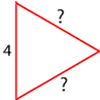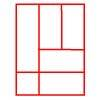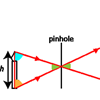Search by Topic

Resources tagged with Similarity and congruence similar to Triangle Transformation:

Filter by: Content type:
Age range:
Challenge level:

There are 9 results

Broad Topics > Angles, Polygons, and Geometrical Proof > Similarity and congruenceOverlap

Age 11 to 14 Challenge Level:

A red square and a blue square overlap so that the corner of the red square rests on the centre of the blue square. Show that, whatever the orientation of the red square, it covers a quarter of the. . . .Tennis

Age 11 to 14 Challenge Level:

A tennis ball is served from directly above the baseline (assume the ball travels in a straight line). What is the minimum height that the ball can be hit at to ensure it lands in the service area?How Big?

Age 11 to 14 Challenge Level:

If the sides of the triangle in the diagram are 3, 4 and 5, what is the area of the shaded square?Triangular Tantaliser

Age 11 to 14 Challenge Level:

Draw all the possible distinct triangles on a 4 x 4 dotty grid. Convince me that you have all possible triangles.Hex

Age 11 to 14 Challenge Level:

Explain how the thirteen pieces making up the regular hexagon shown in the diagram can be re-assembled to form three smaller regular hexagons congruent to each other.Number the Sides

Age 7 to 11 Challenge Level:

The triangles in these sets are similar - can you work out the lengths of the sides which have question marks?Is a Square a Rectangle?

Age 7 to 11 Challenge Level:

How many rectangles can you find in this shape? Which ones are differently sized and which are 'similar'?Same Length

Age 11 to 16 Challenge Level:

Construct two equilateral triangles on a straight line. There are two lengths that look the same - can you prove it?Pinhole Camera

Age 11 to 14 Challenge Level:

Make your own pinhole camera for safe observation of the sun, and find out how it works.